Home > CCA2 > Chapter 8 > Lesson 8.3.2 > Problem8-163

8-163.
1.For homework, Londa was asked to simplify the expression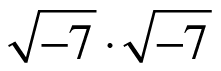. She got the answer 7, but when she checked, she learned that the correct answer was −7. Homework Help ✎

1. Show Londa the steps she could take to get −7.

2. What steps do you think Londa took to get 7 as a result?

3. What does she need to consider to avoid making this mistake in the future?

4. Londa's example means that it is not always true that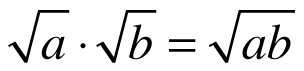for real numbers a and b. What restriction needs to be placed on the numbers a and b?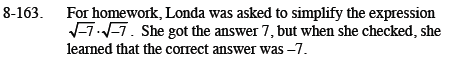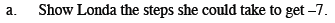$\sqrt{-7} \cdot \sqrt{-7} = i\sqrt{7} \cdot i\sqrt{7}$

$=i^2\sqrt{49}$

$-1\sqrt{49}$

$= -1 \cdot 7 = -7$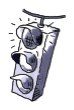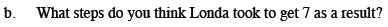Look at the negative signs.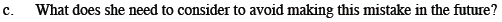Convert to imaginary form before completing operations.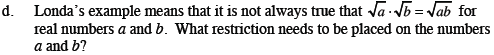They must be non-negative real numbers.